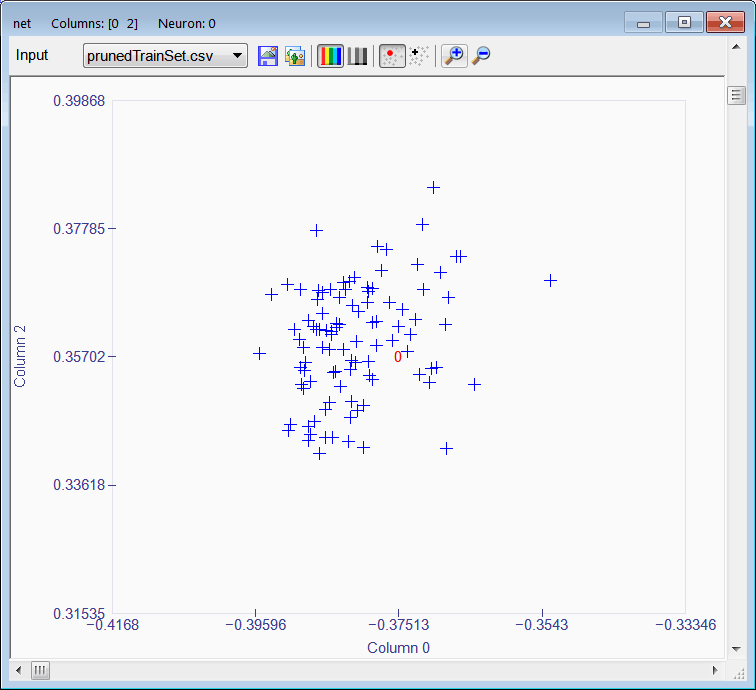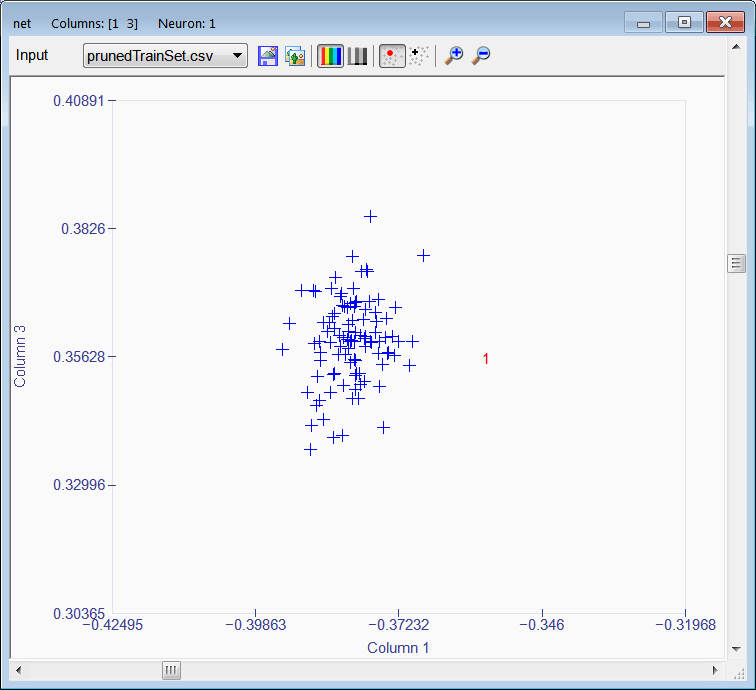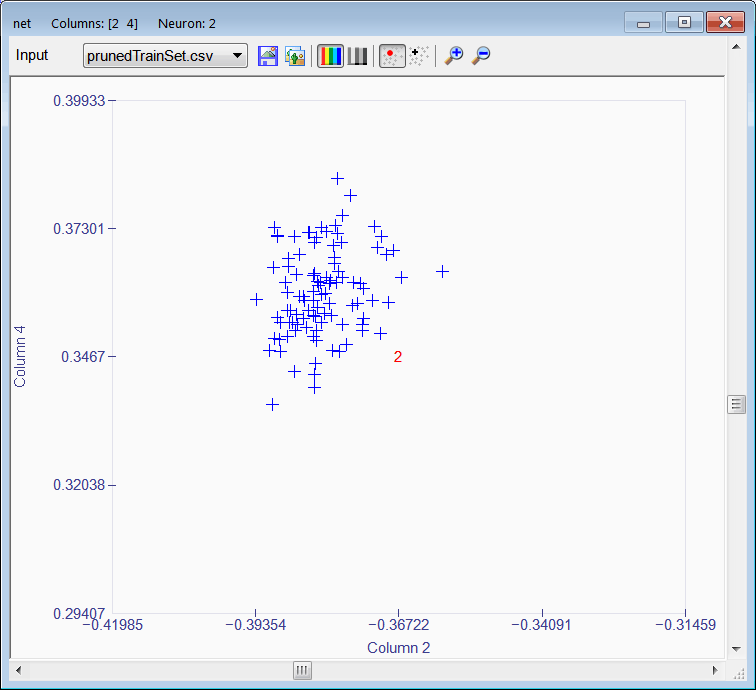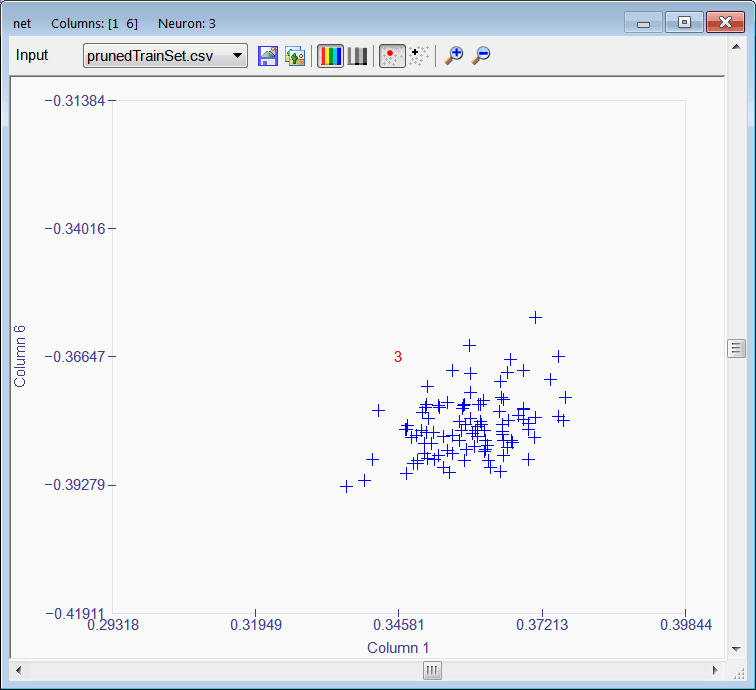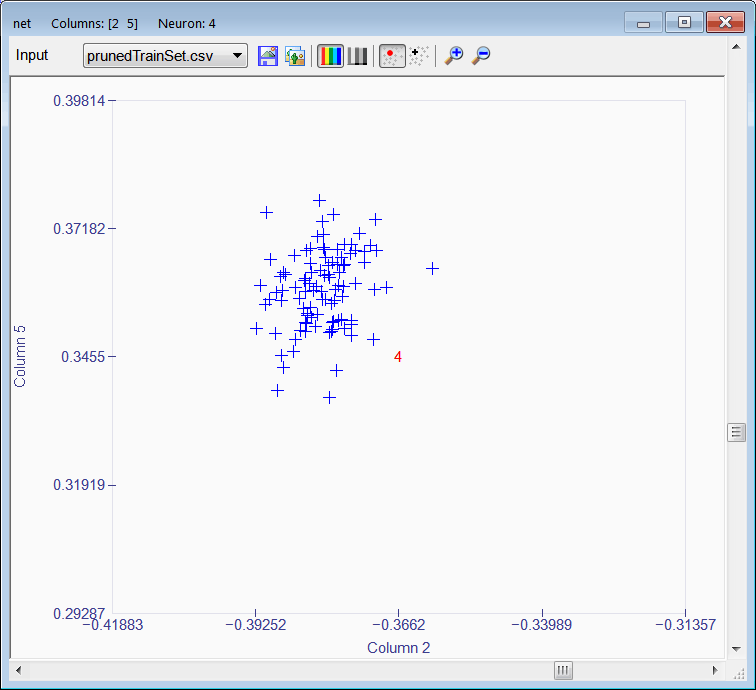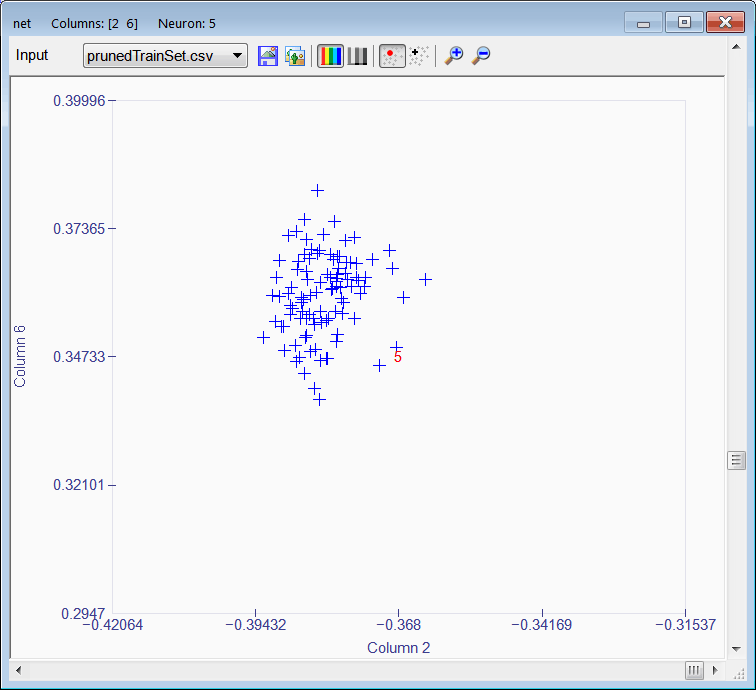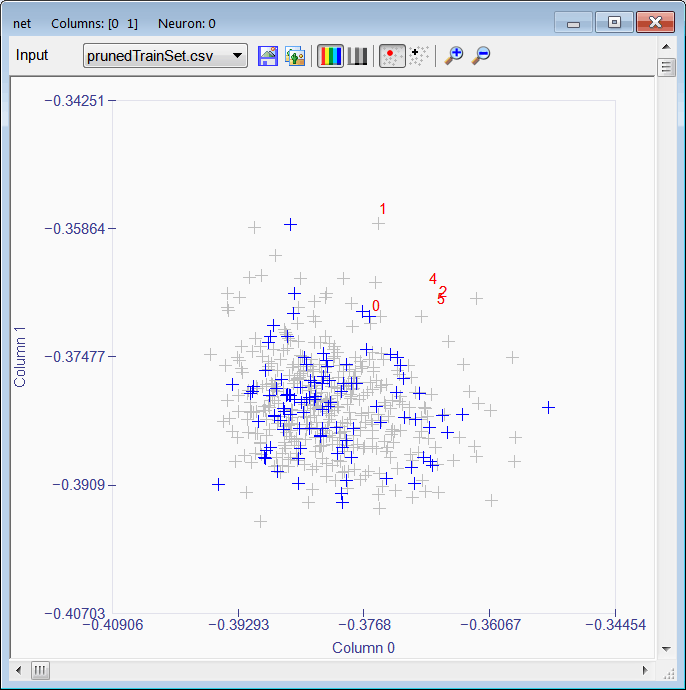# Review

 Problem 1 Create a project called DigitalSystem to classify signals using a Kohonen network. A digital system has a sampling frequency fs = 256 Hz (256 samples per seconds). The system operates with signals of frequency: 4 Hz, 8 Hz, 15 Hz, 22 Hz, 28 Hz and 34 Hz. A student is building a training set input to train a Kohonen network. Each training case has duration of one second. The first 100 cases have a frequency of 4 Hz (four complete cycles per case). The following 100 cases have a frequency of 8 Hz. The last 100 cases have a frequency of 34 Hz. The student is adding 10 % of noise to the cases. Write a program (called BuildTrainSet.lab) to create the trainSetIn.csv file with 256 columns and 600 rows. You may use a random phase to create different cases of the same frequency. The formula below shows the relationship between the continuous time t, and discrete time n.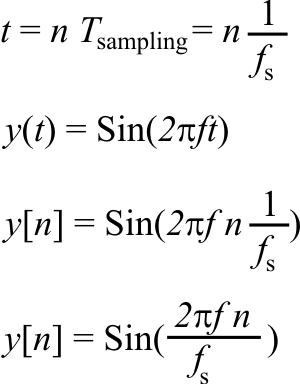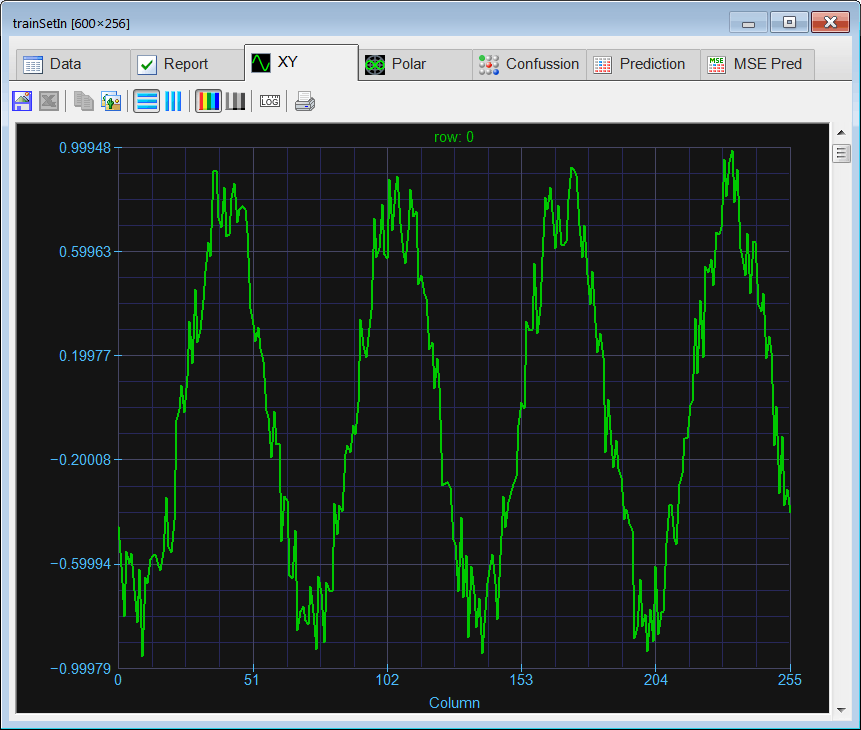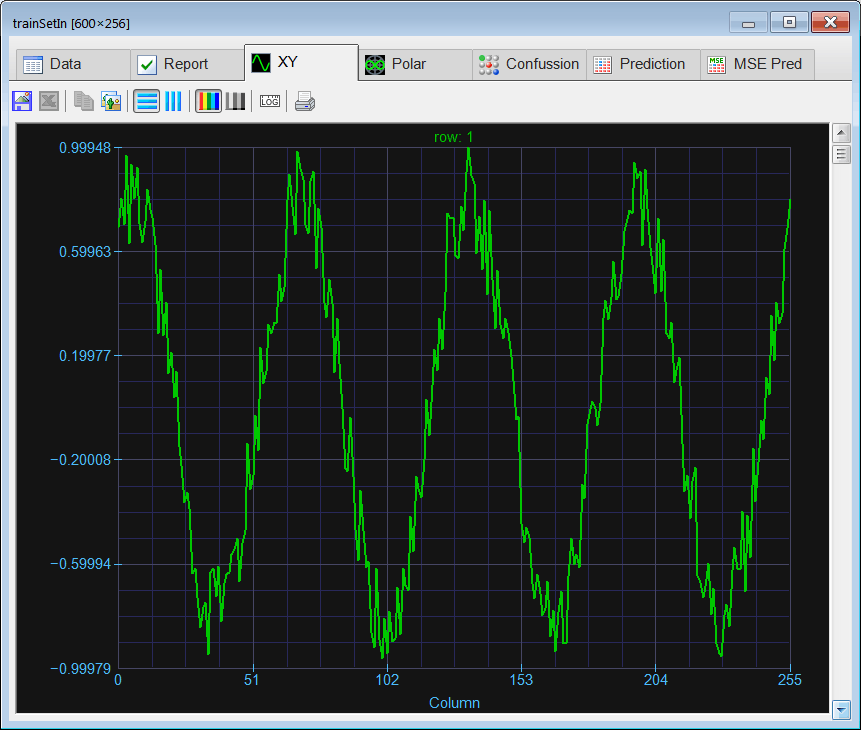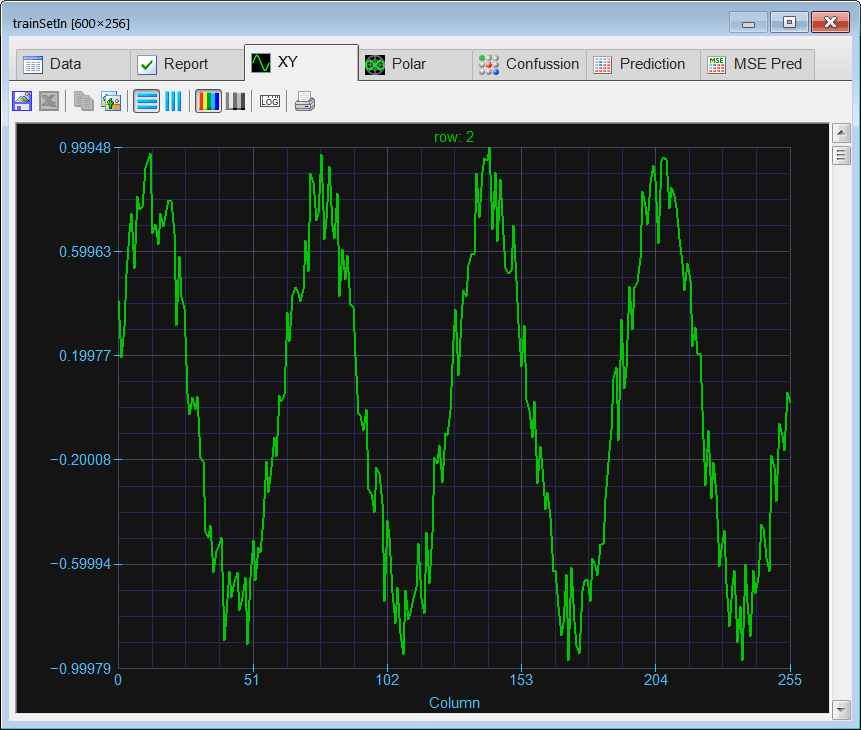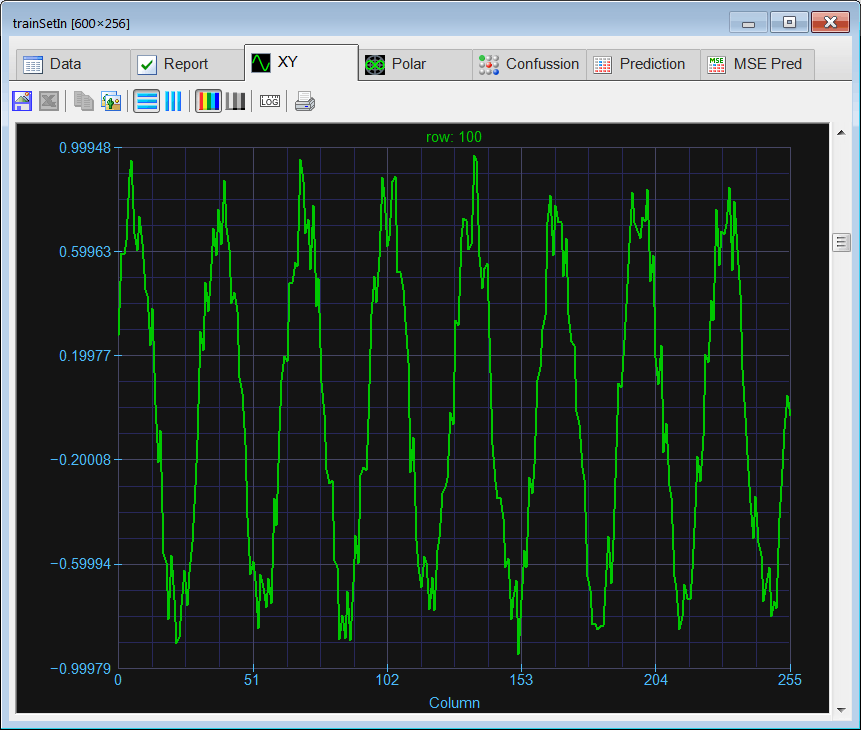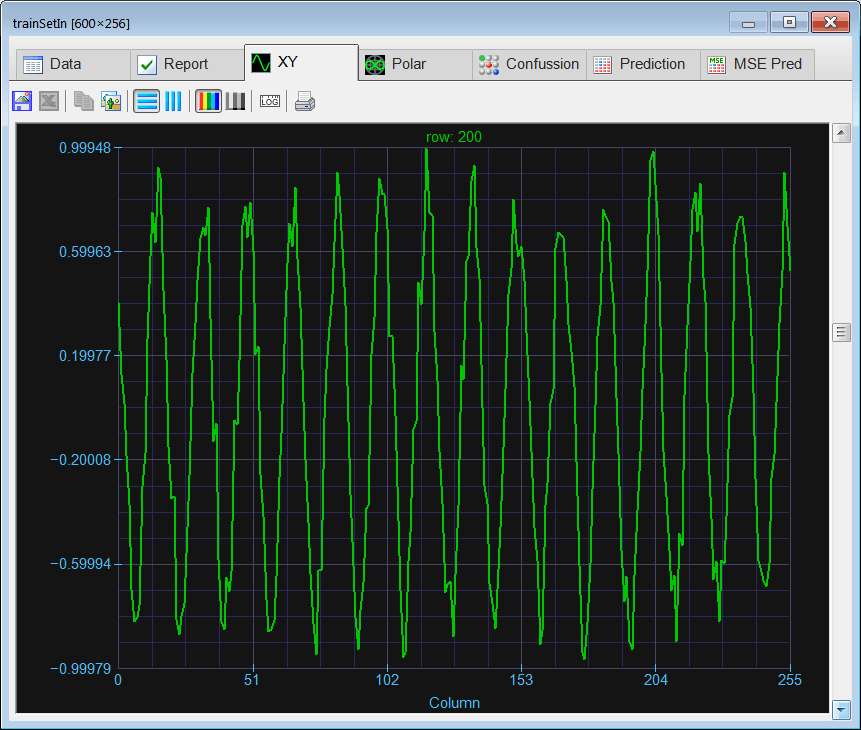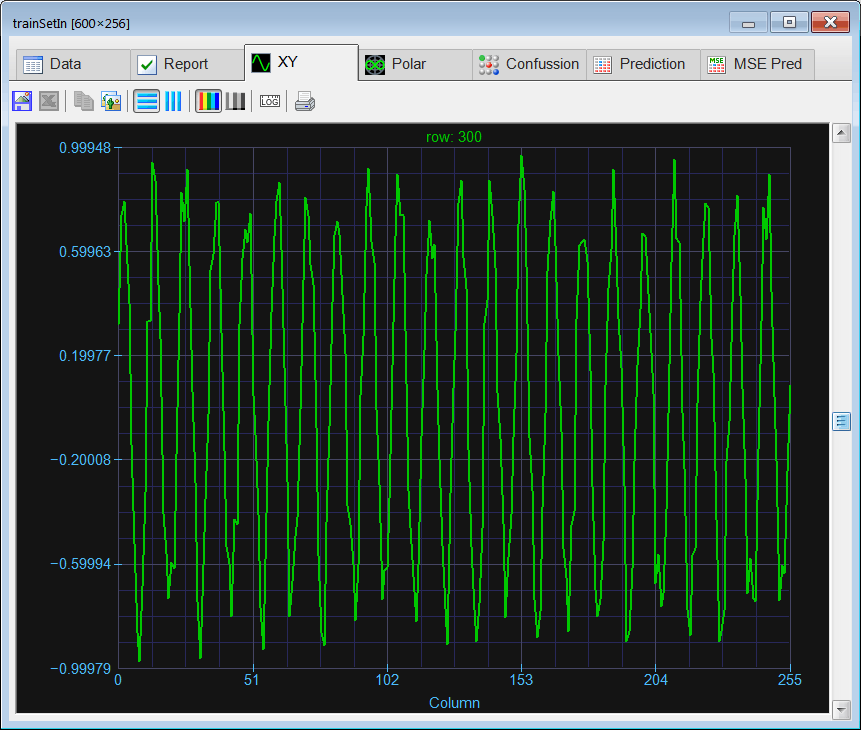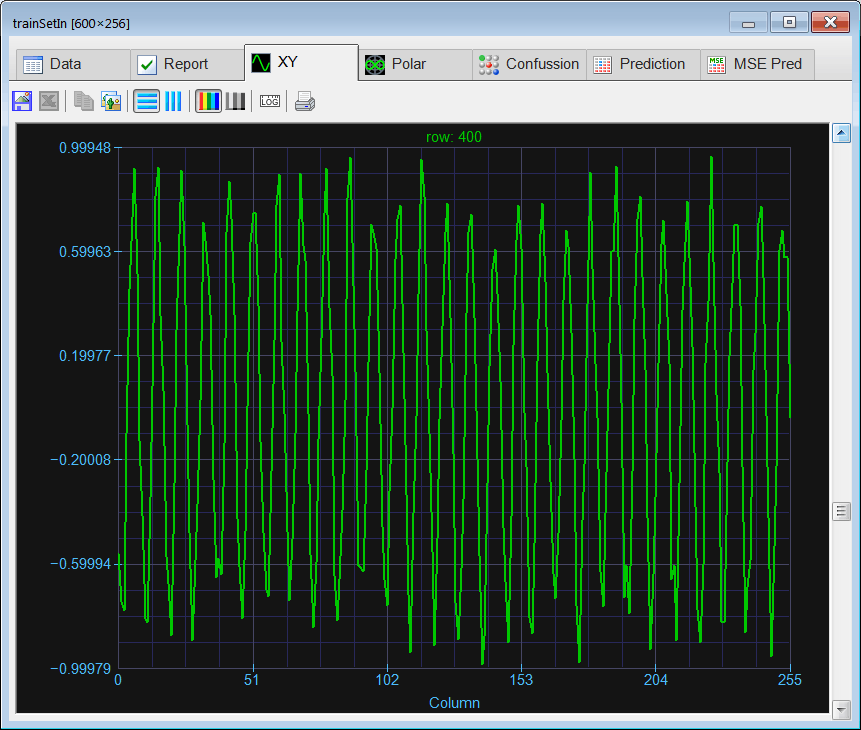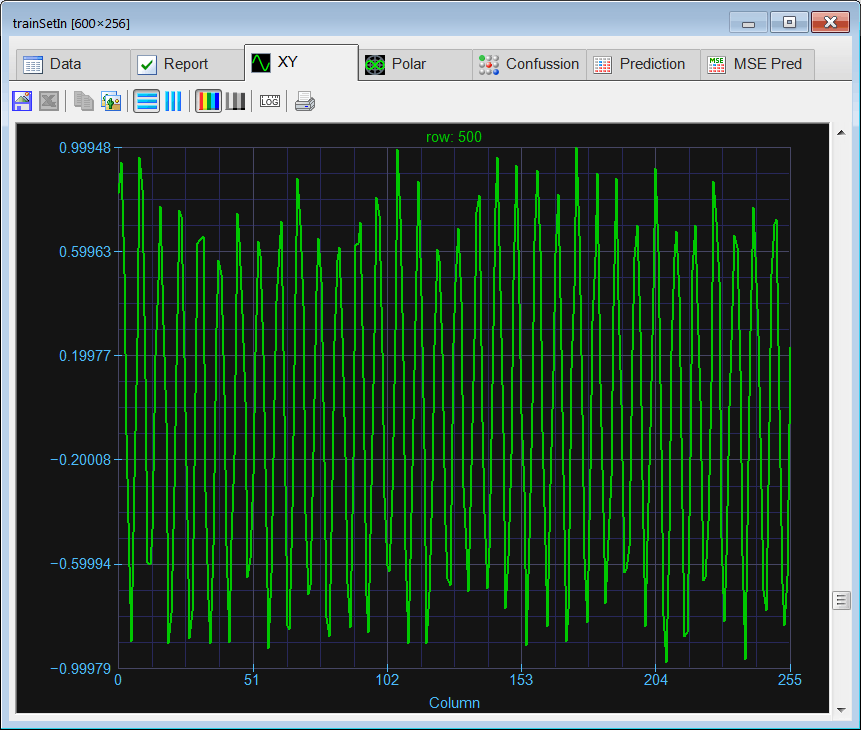Problem 2 As the training set includes periodic signals, we may use the Fourier Transform to try to reduce the number of inputs in the Kohonen network. As phase information is not important, we can use the magnitude of the transformed signal. You may use the spectrum function that returns the magnitude of the Fast Fourier Transform of a signal removing completely phase information. Create the Transform.lab file to transform the data in the trainSeIn.csv file, and create the trainsSetFreq.csv file with the training set input in the frequency domain.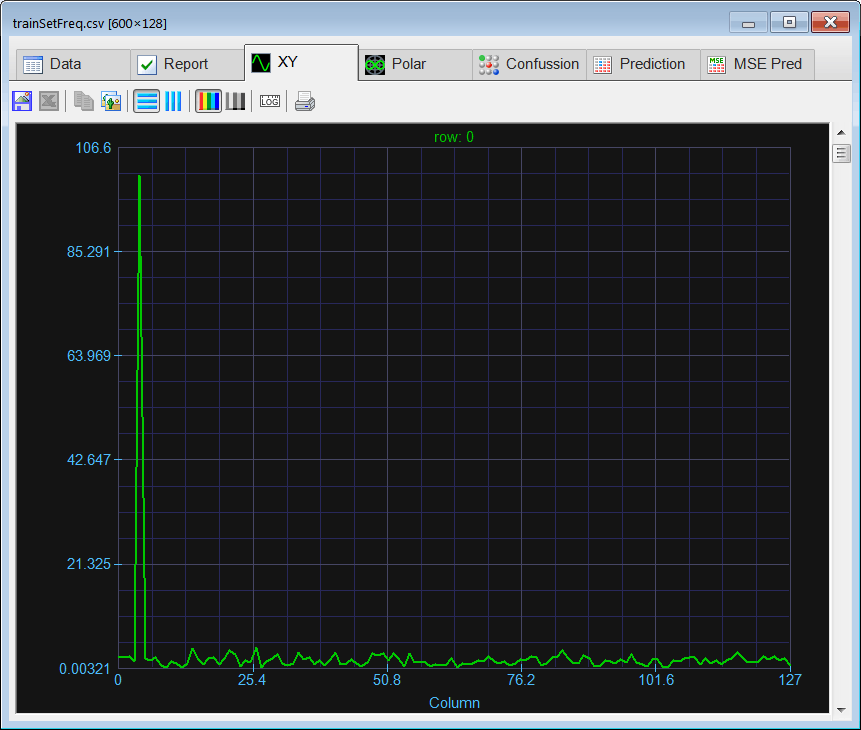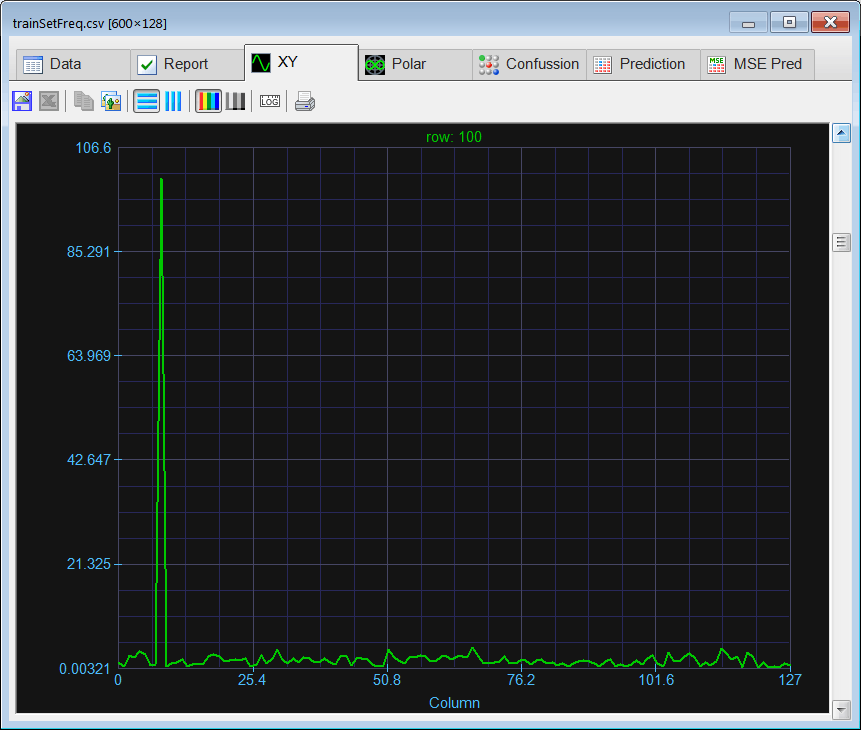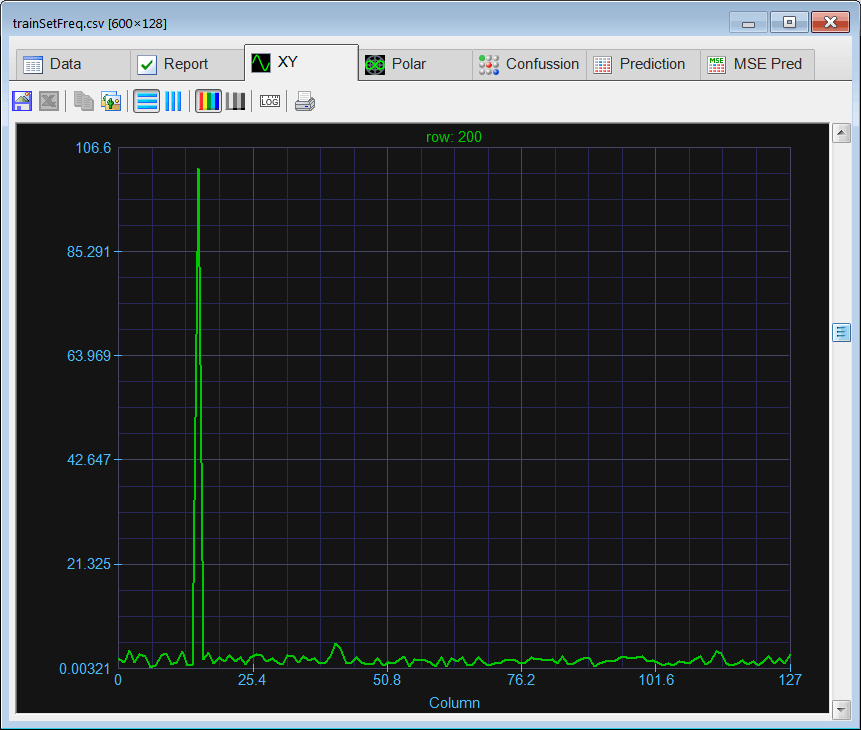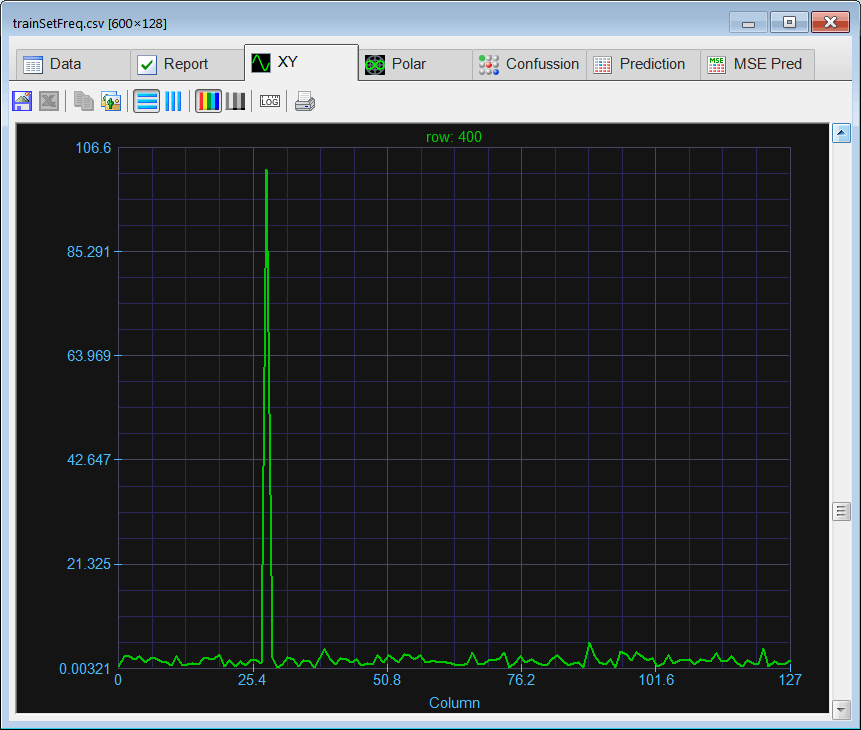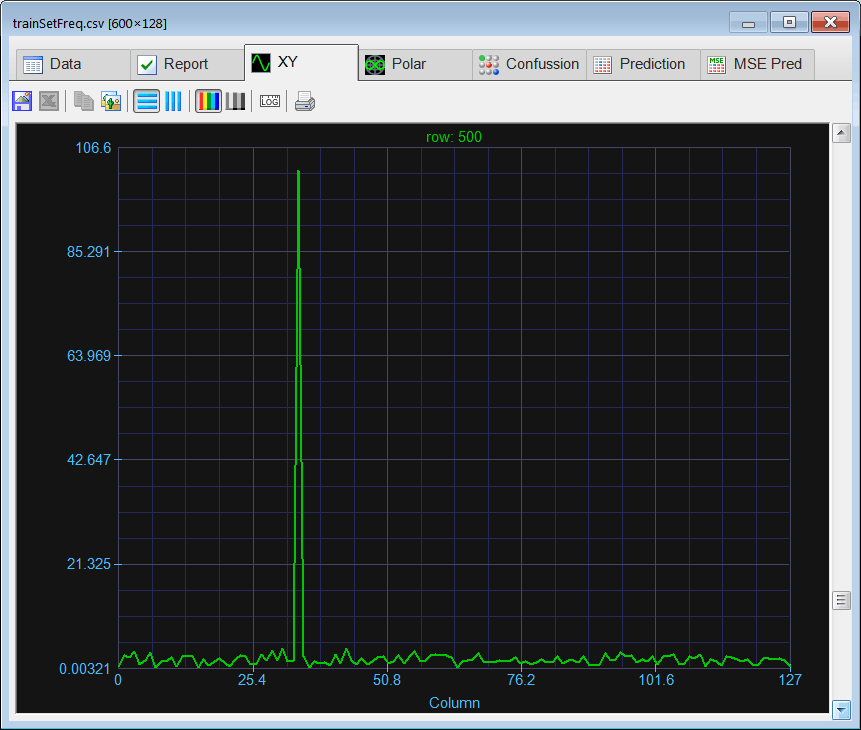Problem 3 Reduce the number of inputs by removing those input variables (columns) with little information. That is those input variable with little variance. Write the Prune.lab file. First, we review the report from the trainSetFreq.csv file. From the report, it can be seen that many components have a standard deviation less than one (variance less than one). Second, we must remove the input variables (columns) that have a variance less than one.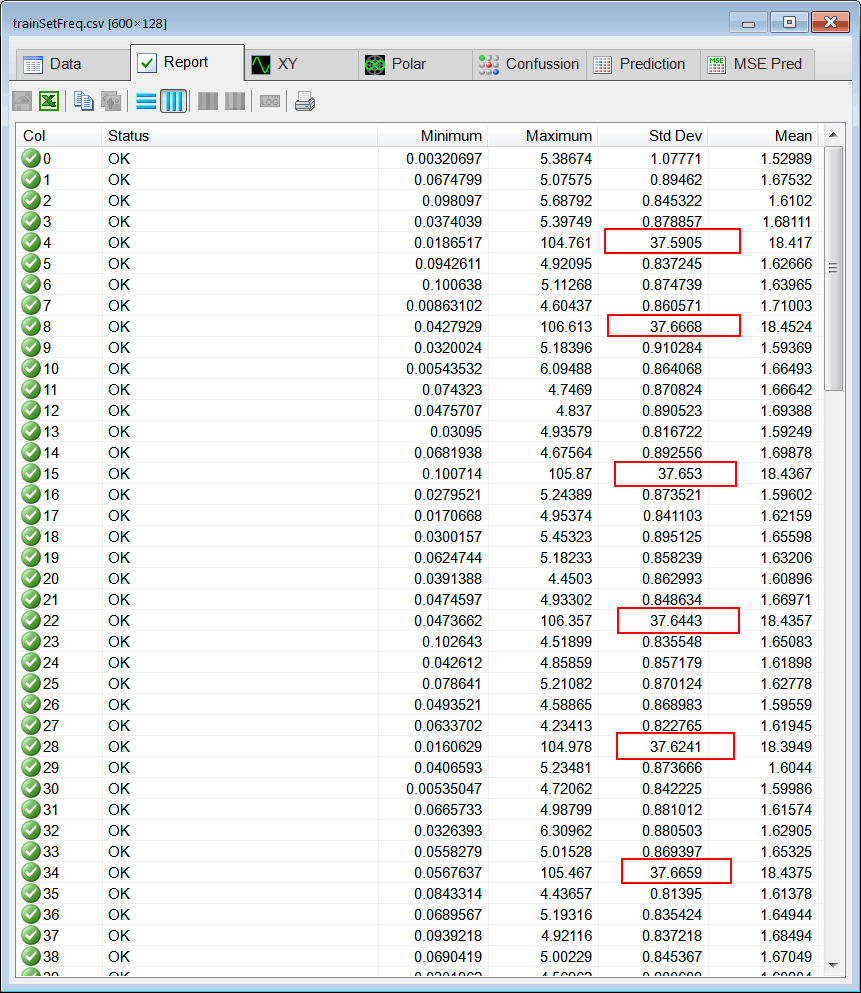DigitalSystem\Prune.lab //______________________________ Load the Input Matrix trainSetFreq; trainSetFreq.Load(); int numRows = trainSetFreq.GetRowCount(); //______________________________ Compute the Variance Vector varianceInput = trainSetFreq.ColsVar(); //______________________________ Compute how many columns we keep int count = 0; ... //____________________________ Find which columns we keep Vector keep; keep.Create(count); ... //_____________________________ Create the Pruned Train Set Matrix prunedTrainSet; prunedTrainSet = trainSetFreq.GetCols(keep); prunedTrainSet.Save();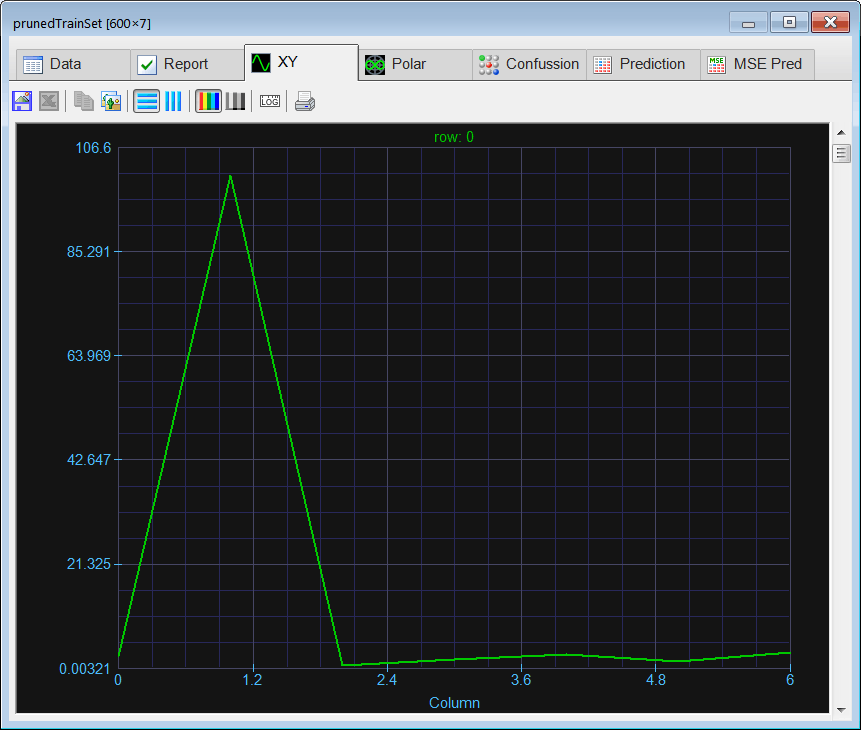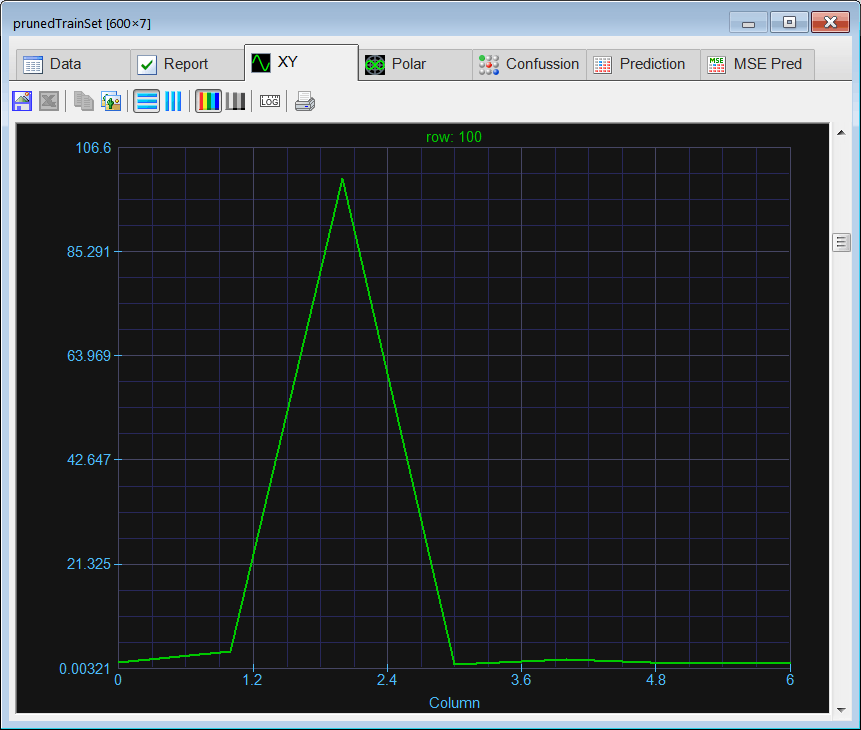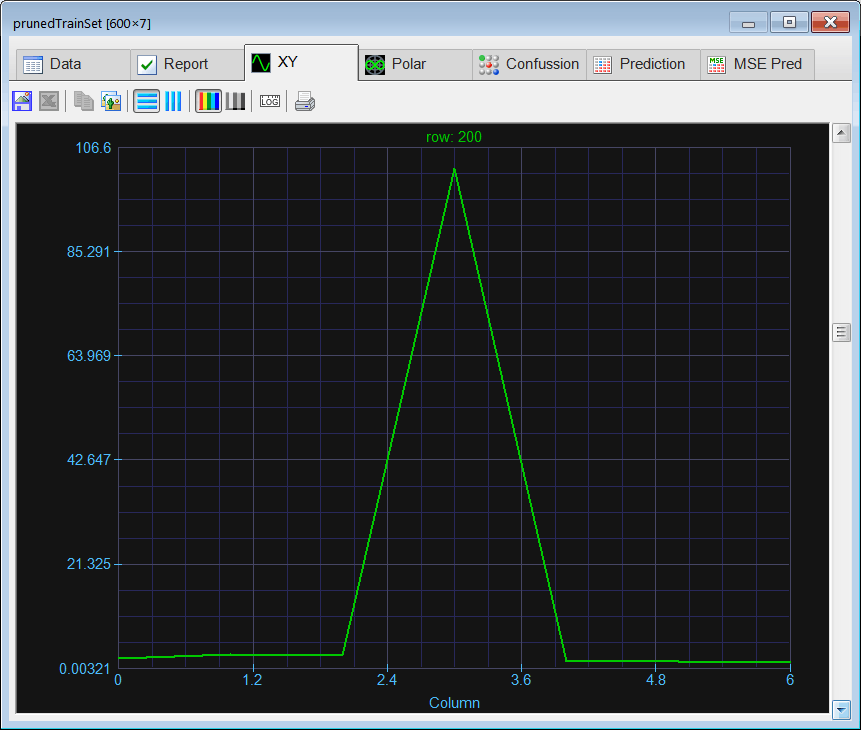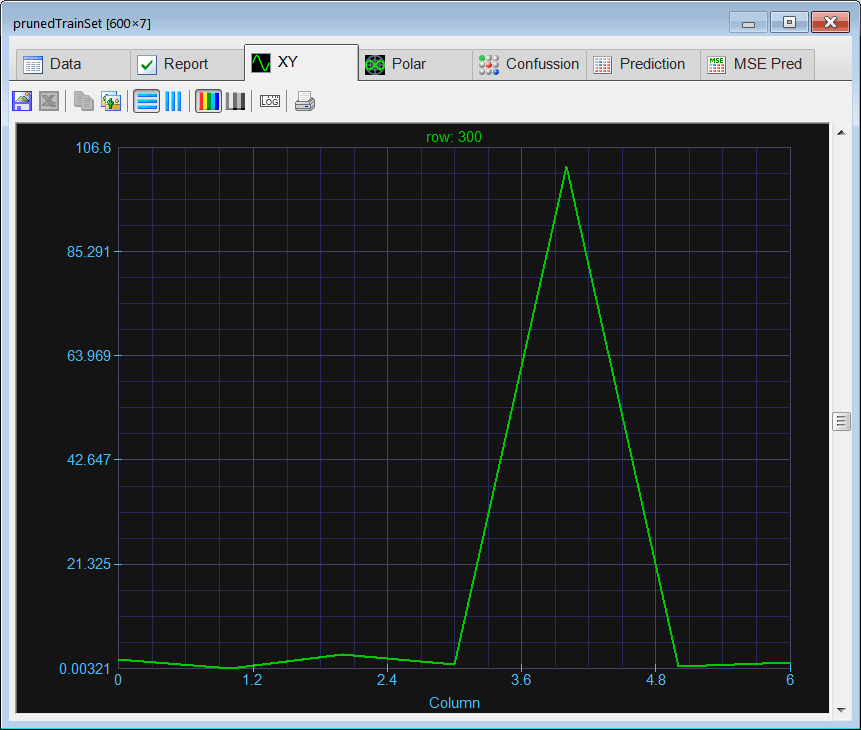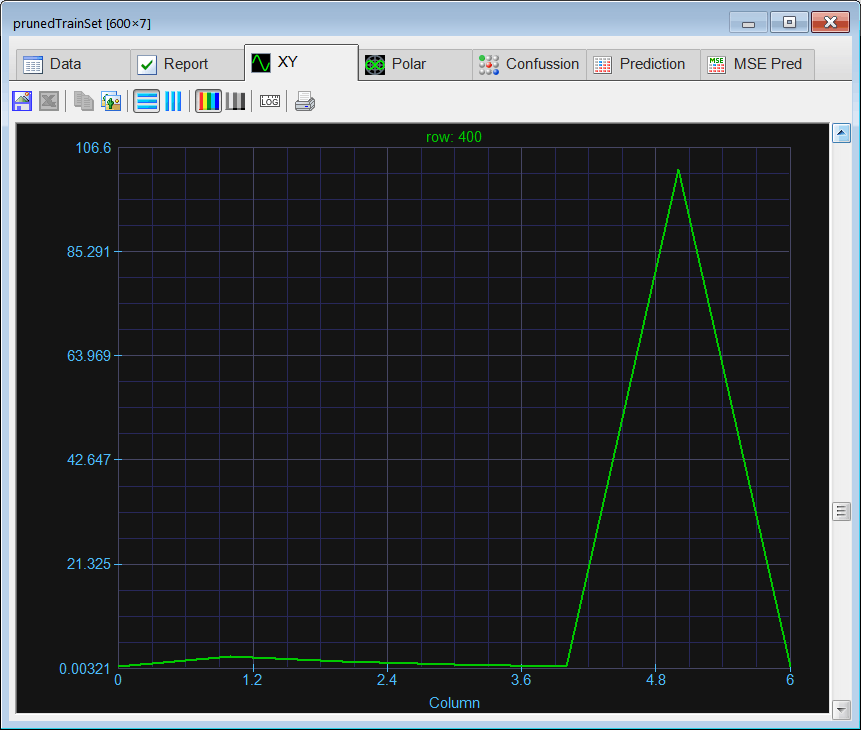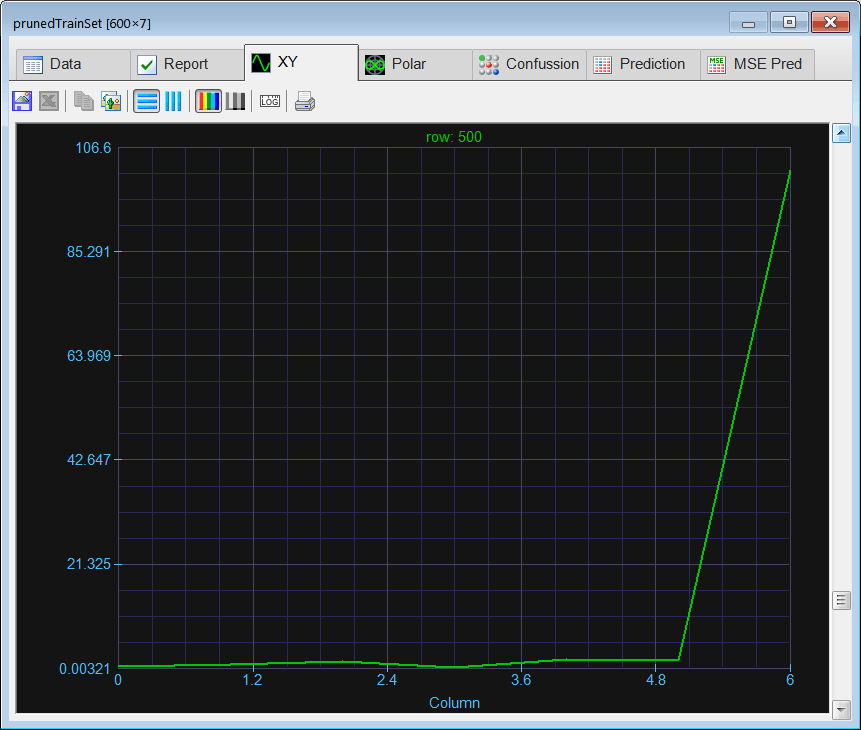Problem 4 Write the Train.lab file to Create and train a Kohonen network to classify the signals (of problem 1) in the frequency domain. Use Multiplicative Input normalization.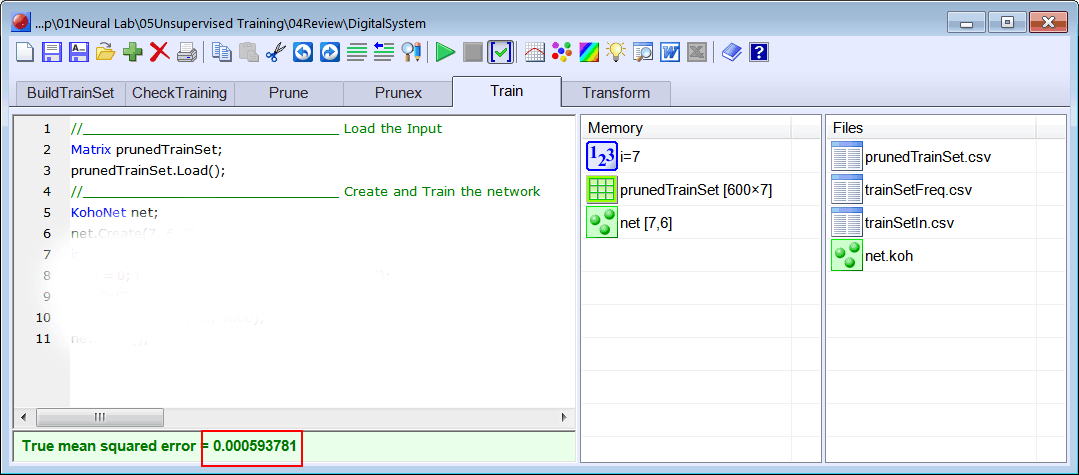Problem 5 Write the CheckTraining.lab file to check the training of the Kohonen network of the previous problem.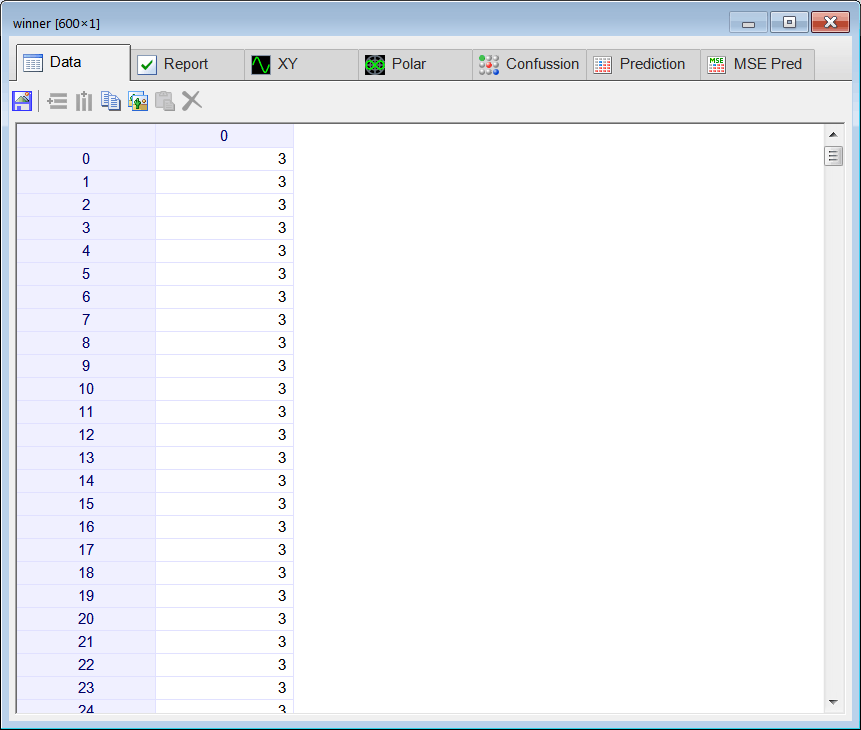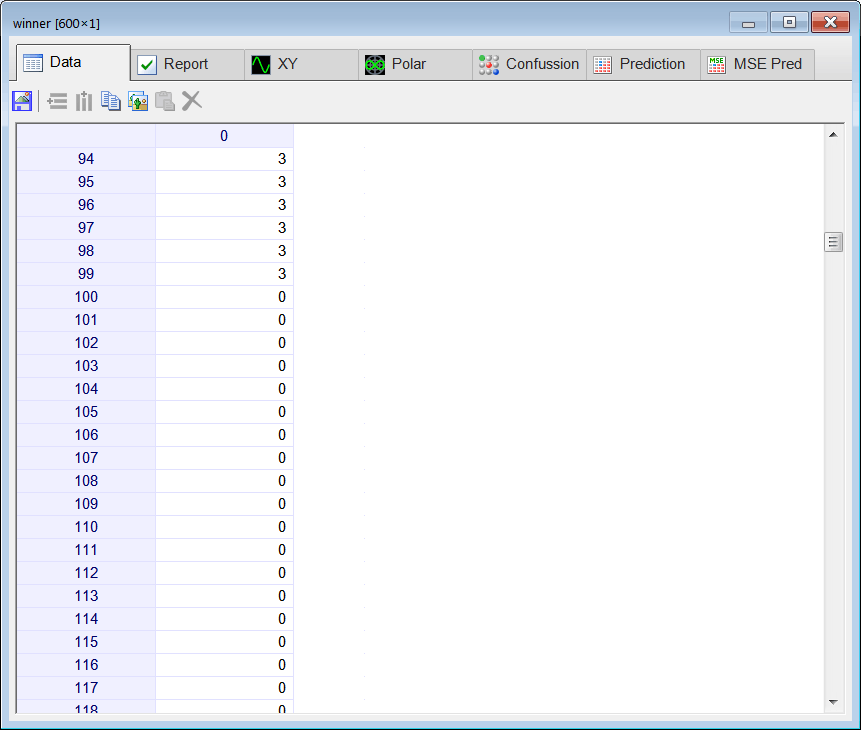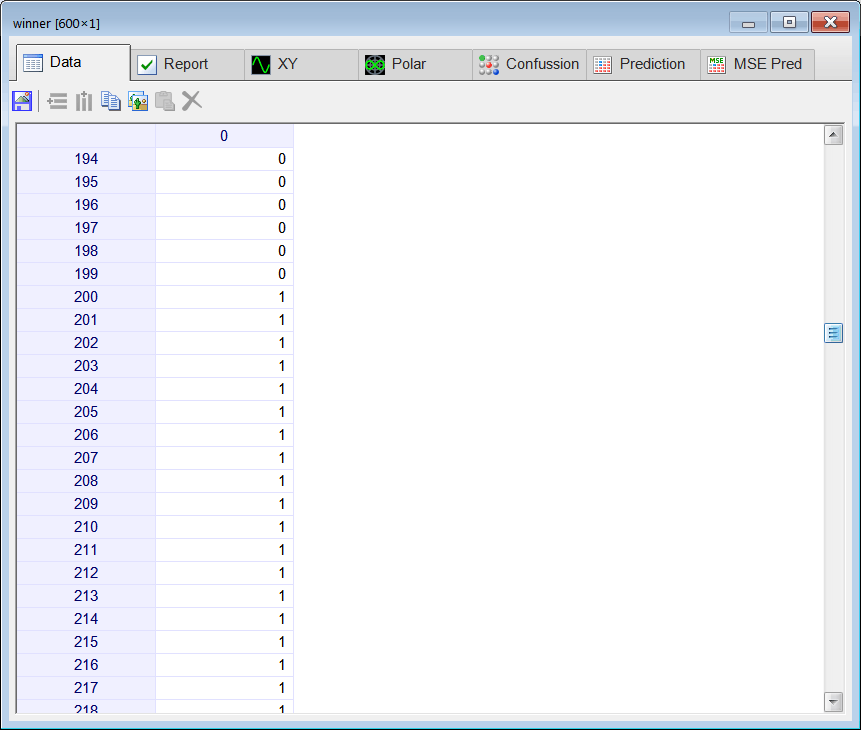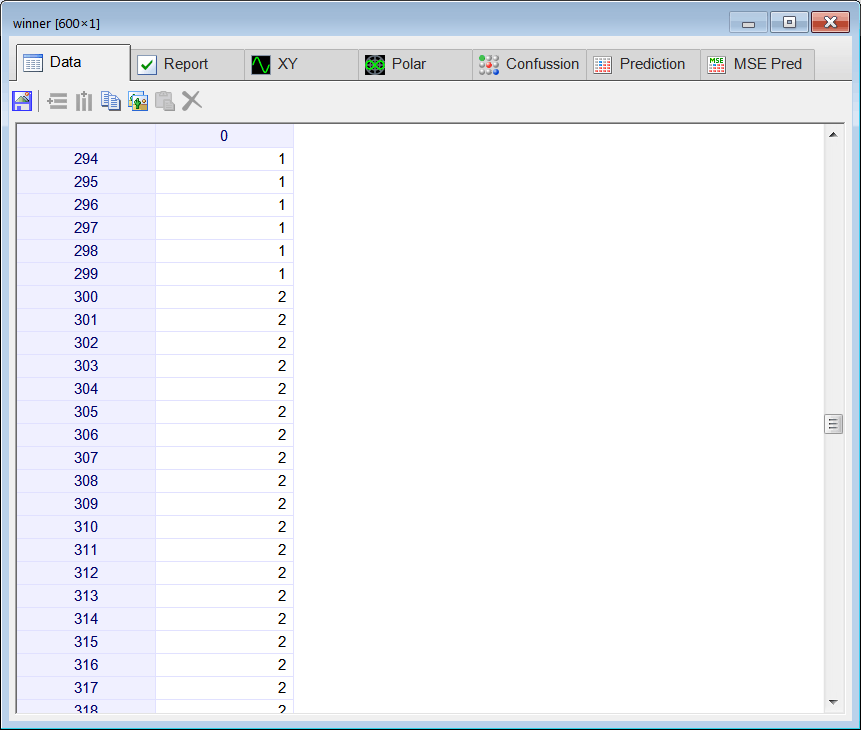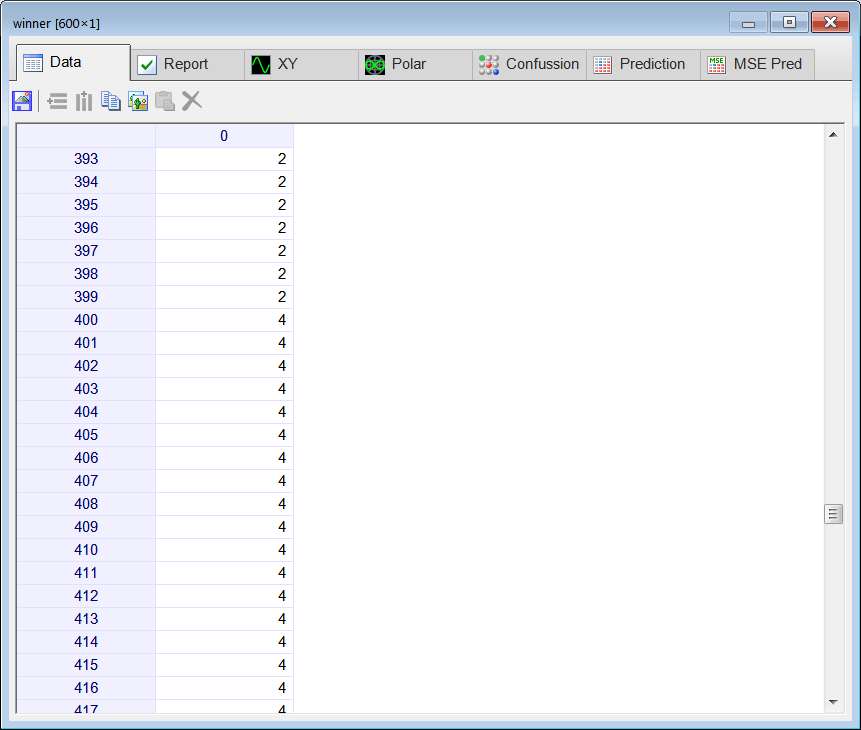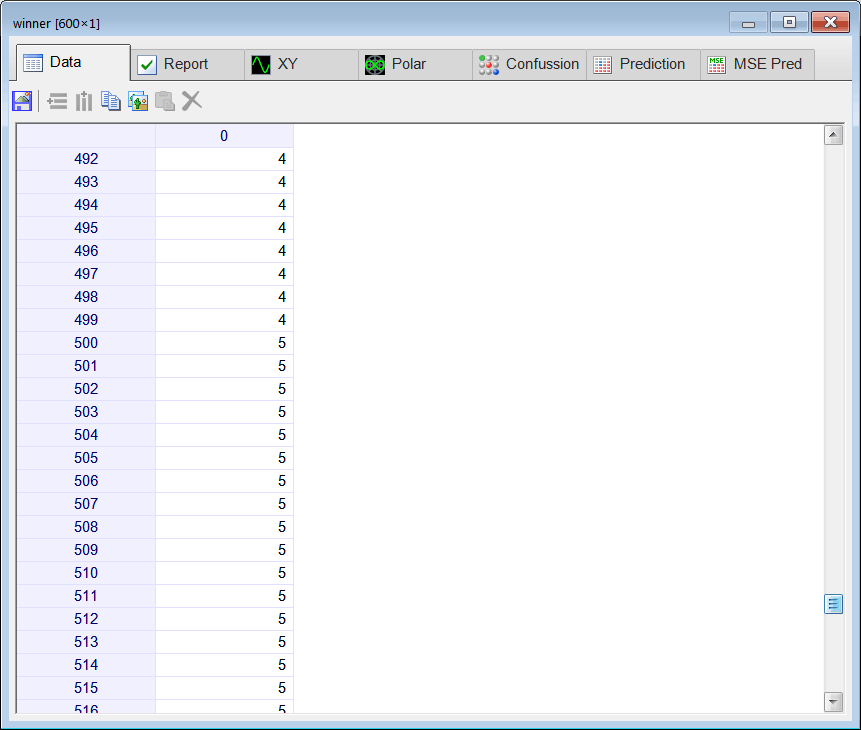Problem 6 Review the behavior of the Kohonen network of problem 3. Write some conclusions about your results.